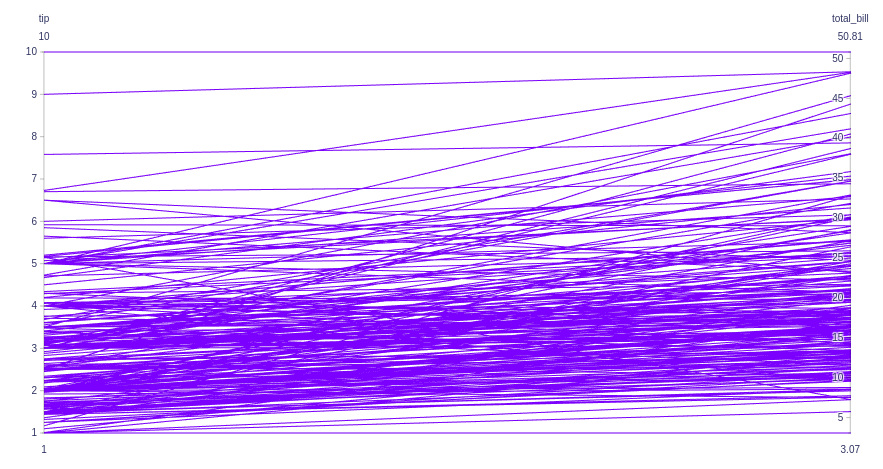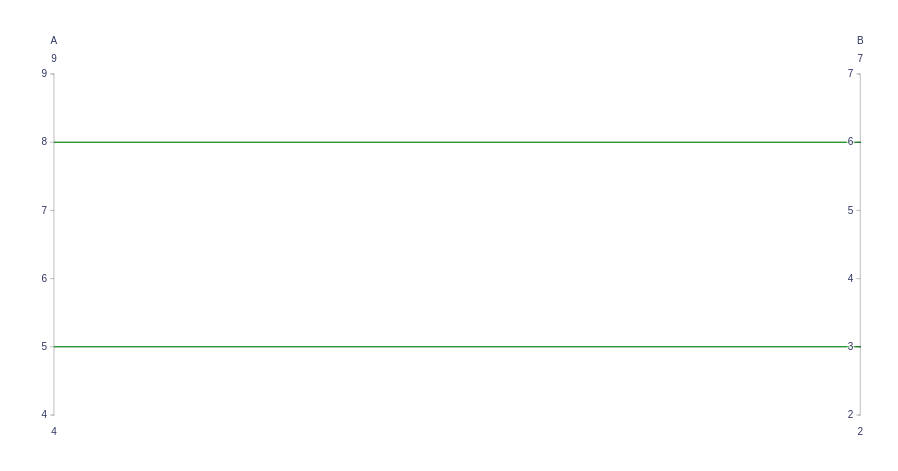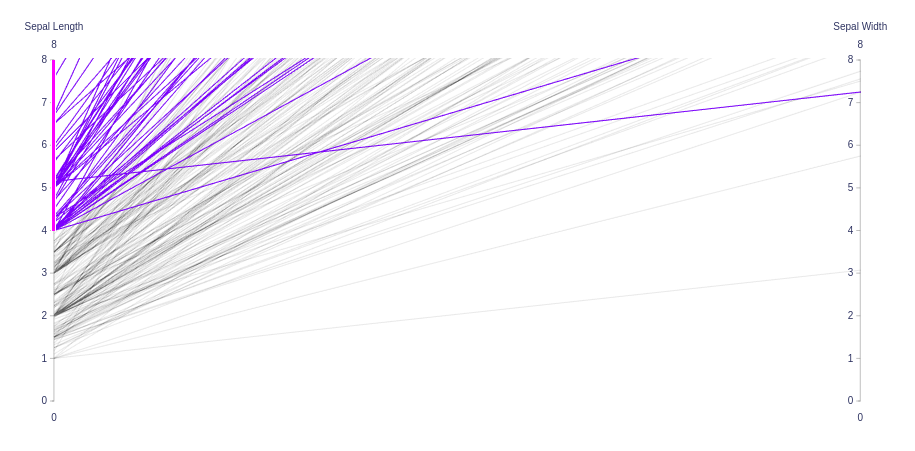# Parallel Coordinates Plot using Plotly in Python

A Plotly is a Python library that is used to design graphs, especially interactive graphs. It can plot various graphs and charts like histogram, barplot, boxplot, spreadplot, and many more. It is mainly used in data analysis as well as financial analysis. Plotly is an interactive visualization library.

## Parallel Coordinates Plot

Parallel coordinates plot is a common way of visualizing and analyzing high-dimensional datasets. A point in n-dimensional space is represented as a polyline with vertices on the parallel axes and the position of the vertex corresponds to the coordinate of the point.

Syntax: parallel_coordinates(data_frame=None, dimensions=None, labels={}, range_color=None)

Parameters:

data_frame: This argument needs to be passed for column names (and not keyword names) to be used.

dimensions: Either names of columns in data_frame, or pandas Series, or array_like objects Values from these columns are used for multidimensional visualization.

labels: By default, column names are used in the figure for axis titles, legend entries and hovers. This parameter allows this to be overridden. The keys of this dict should correspond to column names, and the values should correspond to the desired label to be displayed.

range_color: If provided, overrides auto-scaling on the continuous color scale.

Example 1:

## Python3

 `import` `plotly.express as px ` ` `  ` `  `df ``=` `px.data.tips() ` `fig ``=` `px.parallel_coordinates( ` `    ``df, dimensions``=``[``'tip'``, ``'total_bill'``, ``'day'``,``'time'``],) ` ` `  `fig.show()`

Output:Example 2: Showing Parallel Coordinates Chart with go.Parcoords()

## Python3

 `import` `plotly.graph_objects as go ` ` `  `fig ``=` `go.Figure(data``=``go.Parcoords( ` `    ``line_color``=``'green'``, ` `    ``dimensions``=``list``([ ` `        ``dict``(``range``=``[``4``, ``9``], ` `             ``label``=``'A'``, values``=``[``5``, ``8``]), ` `        ``dict``(``range``=``[``2``, ``7``], ` `             ``label``=``'B'``, values``=``[``3``, ``6``]), ` `    ``]) ` `) ` `) ` ` `  `fig.show() `

Output:Example 3:

## Python3

 `import` `plotly.graph_objects as go ` `import` `plotly.express as px ` ` `  `df ``=` `px.data.tips() ` ` `  `fig ``=` `go.Figure(data``=``go.Parcoords( ` `    ``dimensions``=``list``([ ` `        ``dict``(``range``=``[``0``, ``8``], ` `             ``constraintrange``=``[``4``, ``8``], ` `             ``label``=``'Sepal Length'``, values``=``df[``'tip'``]), ` `        ``dict``(``range``=``[``0``, ``8``], ` `             ``label``=``'Sepal Width'``, values``=``df[``'total_bill'``]), ` `    ``]) ` `) ` `) ` ` `  ` `  `fig.show() `

Output:Attention geek! Strengthen your foundations with the Python Programming Foundation Course and learn the basics.

To begin with, your interview preparations Enhance your Data Structures concepts with the Python DS Course.

My Personal Notes arrow_drop_upIf you like GeeksforGeeks and would like to contribute, you can also write an article using contribute.geeksforgeeks.org or mail your article to contribute@geeksforgeeks.org. See your article appearing on the GeeksforGeeks main page and help other Geeks.

Please Improve this article if you find anything incorrect by clicking on the "Improve Article" button below.

Article Tags :

Be the First to upvote.

Please write to us at contribute@geeksforgeeks.org to report any issue with the above content.